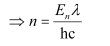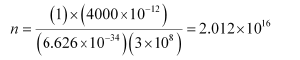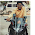## Tuesday, 2 July 2013

### What is the number of photons of light with a wavelength of 4000 pm that provide 1 J of energy?

Question 2.8: What is the number of photons of light with a wavelength of 4000 pm that provide 1 J of energy?
Energy (E) of a photon = hν
Energy (En) of ‘n’ photons = nhνWhere,
λ = wavelength of light = 4000 pm = 4000 ×10-12 m
c = velocity of light in vacuum = 3 × 108 m/s
h = Planck’s constant = 6.626 × 10-34 Js
Substituting the values in the given expression of n:Hence, the number of photons with a wavelength of 4000 pm and energy of 1 J are
2.012 × 1016.

1.2.3.4.Nice attempt.

5.Nice attempt.

6.7.8.